### IMO Shortlist 1999 problem C7

Kvaliteta:
Avg: 0,0
Težina:
Avg: 9,0
Let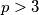$p >3$ be a prime number. For each nonempty subset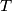$T$ of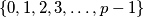$\{0,1,2,3, \ldots , p-1\}$, let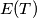$E(T)$ be the set of all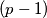$(p-1)$-tuples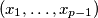$(x_1, \ldots ,x_{p-1} )$, where each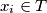$x_i \in T$ and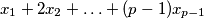$x_1+2x_2+ \ldots + (p-1)x_{p-1}$ is divisible by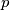$p$ and let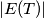$|E(T)|$ denote the number of elements in$E(T)$. Prove that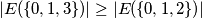with equality if and only if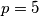$p = 5$.
Izvor: Međunarodna matematička olimpijada, shortlist 1999